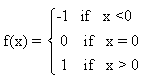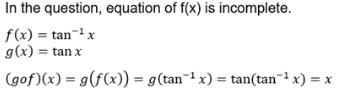Courses

# Test: Composition Of Functions

## 10 Questions MCQ Test Mathematics (Maths) Class 12 | Test: Composition Of Functions

Description
This mock test of Test: Composition Of Functions for JEE helps you for every JEE entrance exam. This contains 10 Multiple Choice Questions for JEE Test: Composition Of Functions (mcq) to study with solutions a complete question bank. The solved questions answers in this Test: Composition Of Functions quiz give you a good mix of easy questions and tough questions. JEE students definitely take this Test: Composition Of Functions exercise for a better result in the exam. You can find other Test: Composition Of Functions extra questions, long questions & short questions for JEE on EduRev as well by searching above.
QUESTION: 1

### Let f: A → B and g : B → C be one - one functions. Then, gof: A → C is

Solution:

We are given that functions f : A→B and g : B→C are both one-to-one functions.
Suppose a1, a2 ∈ A such that (gof)(a1) = (gof)(a2)
⇒ g(f(a1)) = g(f(a2)) (definition of composition) Since g is one-to-one, therefore,
f(a1) = f(a2)
And since f is one-to-one, therefore, a1 = a2
Thus, we have shown that if (gof)(a1) = (gof)(a2) then a1 = a2
Hence, gof is one-to-one function.

QUESTION: 2

### If f : A → B and g : B → C be two functions. Then, composition of f and g, gof : A → C is defined a​

Solution:

A composite function is denoted by (g o f) (x) = g (f(x)). The notation g o f is read as “g of f”.
Example : Consider the functions f: A → B and g: B → C. f = {1, 2, 3, 4, 5}→ {1, 4, 9, 16, 25} and g = {1, 4, 9, 16, 25} → {2, 8, 18, 32, 50}. A = {1, 2, 3, 4, 5}, B = {16, 4, 25, 1, 9}, C = {32, 18, 8, 50, 2}.Here, g o f = {(1, 2), (2, 8), (3, 18), (4, 32), (5, 50)}.

QUESTION: 3

### f: R → R is defined by  f(x) = x2 - 2x + 1. Find f[f(x)]

Solution:
QUESTION: 4

Let f: Q→Q be a function given by f(x) = x2,then f -1(9) =​

Solution:

If f : A → B such that y implies B then f-1(y) = {x implies A : f(x) = y}
Let f-1(9) = x
f(x) = 9
x2 = 9
x = +-3
Thus, f-1(9) = {-3,3}

QUESTION: 5

Let  g(x) = 1 + x – [x] andThen f{g(x)} for all x, is equal to:

Solution:

g(x) = 1 + x - [x]
f(x) = {-1, x<0   0, x=0    1, x>0}
f(g(x)) = f[1 + x - [x]]
= f[1 + x - 1]
= f(x)
f(g(x)) is equal to f(x)

QUESTION: 6

If ƒ(x) = xsecx, then ƒ(0) =

Solution:

f(0)=0×sec0
f(0)=0×1
f(0)=0

QUESTION: 7

Let f = {(1, 3), (2, 1), (3, 2)} and g = {(1, 2), (2, 3), (3, 1)}. What is gof(2)?

Solution:

gof(2)=g[f(2)]
=g=2

QUESTION: 8

If ƒ(x) = tan-1 x and g(x) = tan(x), then (gof)(x) =

Solution:QUESTION: 9

If f(x) = |x| and g(x) = |5x – 2|. Then, fog =​

Solution:

f(x) = |x|   g(x) = |5x - 2|
fog = f(g(x) = g(x) = |5x - 2|

QUESTION: 10

If f: R → R and g: R → R defined by f(x) = 2x + 3 and g(x) = x2 + 7, then the value of x for which f(g(x)) = 25 is

Solution:

f : R → R     g : R → R
f(g) : R → R
f(x) = 2x + 3      g(x2) = x2 + 7
f(g(x)) = 2g(x) + 3
f(g(x)) = 2(x2 + 7) + 3
= 2x2 + 17
As it is given f(g(x)) = 25
Comparing both the eq, we get
2x2 + 17 = 25
2x2 = 8
x = +